# Calculation of the length of a simple pendulum

in StemSocial4 months ago (edited)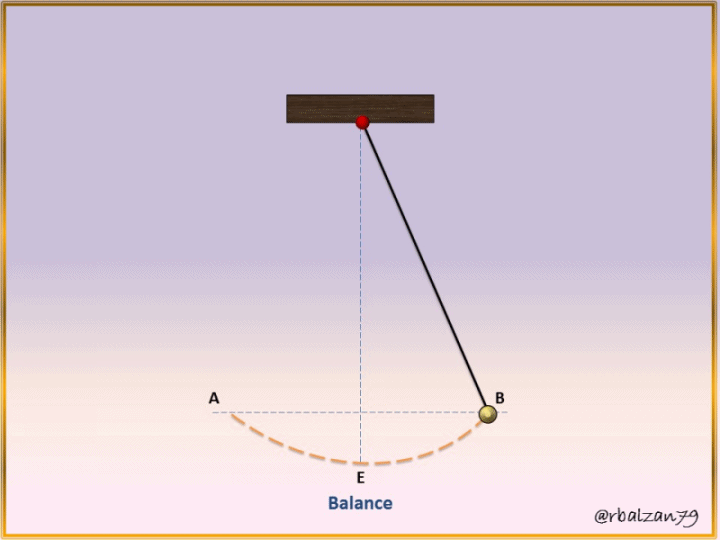Greetings again my dear friends I hope you are all very well, this time we will relate to the movement of a simple pendulum, one that has a point mass (m), which we tie or hold a thread length (L) and, the mass can make it swing in relation to its vertical stable equilibrium point, of course, holding or tying the other end of the thread that holds the mass, as you can see or observe in the following figure 1.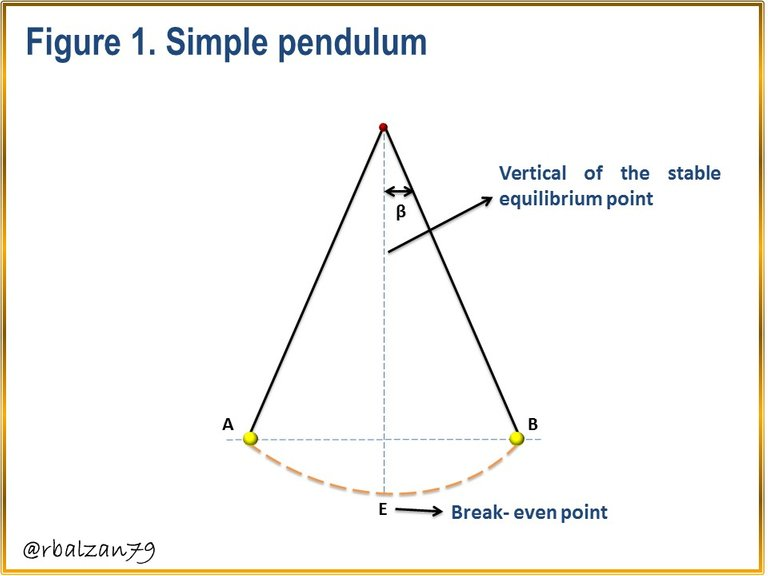We can also express that the simple pendular movement can be considered as a simple harmonic movement (especially for those angles of small oscillations), remember that a simple harmonic movement is one which oscillates periodically from one side to another, that is, a back and forth movement in relation to its stable equilibrium point, highlighting that in the pendular movement, the force that works is not elastic, but gravitational, as you could notice in Figure 1.

Exercise

With the purpose of knowing some values related to the simple pendulum movement, a student proposes to time the respective oscillations of a certain simple pendulum, so that after 20 seconds, the student counts 35 complete oscillations, therefore, in relation to the above, answer the following questions:

a.- What will be the frequency of these oscillations?
b.- What will be the Period of the oscillations?
b.- What will be the length of such a simple pendulum?

Solution

Data:
t = 20 s (Timed time).
Cycles = 35
g = 9,8 m/s2 (Gravity acceleration).
f = ? (Frequency of oscillations).
P = ? (Period of oscillations).
L = ? (Simple pendulum length).

a.- To start our first answer, we must express that the magnitude of the frequency of the oscillation is represented by the number of such oscillations carried in one second, thus we have the following formula 1.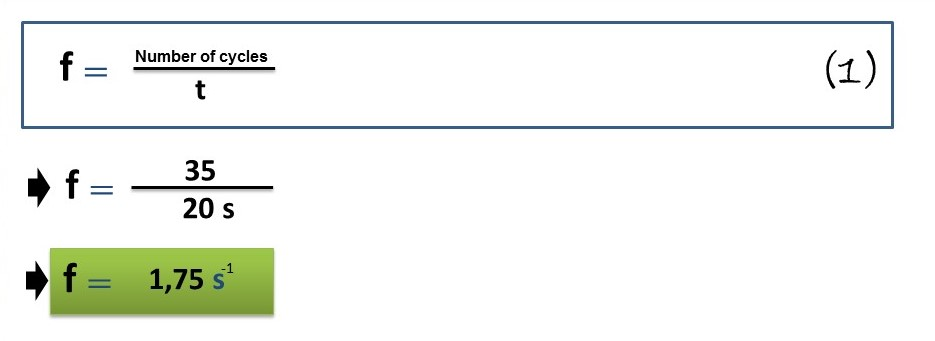With the above relation we can calculate the frequency of oscillation carried out by the simple pendulum which is 1.75 oscillations per second.

b.- With this value we can move on to answer our second question, that is, the calculation of the period of oscillations (T), and we know that this value is the inverse of the frequency already obtained, therefore: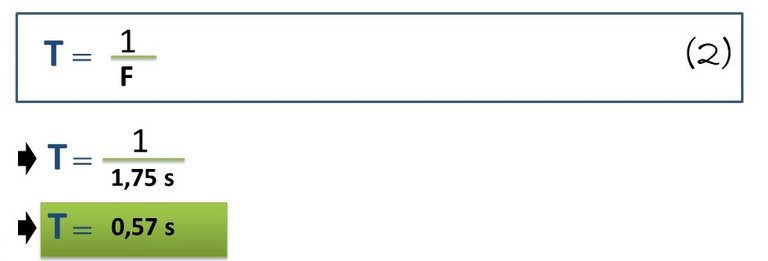Thus, we determine that the pendulum completes one oscillation every 0.57 sec.

c.- Now with this value we can move on to our third question, that is, the calculation of the length (L) of the simple pendulum, for this we will use the following formula: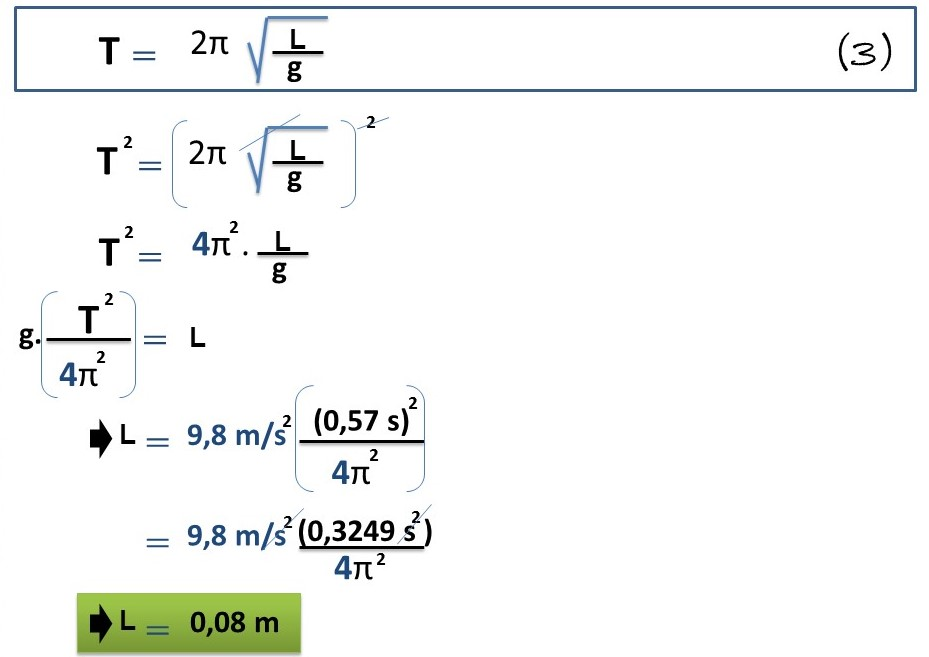In this way we were able to obtain the length of the simple pendulum used by the student to know each of the previous values such as, frequency of oscillation (f), period of oscillation (T) and, the value indicated above of the length of the simple pendulum (L).

# Analysis of results

Innumerable are the movements that develop around us, as the one shown in this opportunity that of the simple pendulum, highlighting characteristics of the period of a simple pendulum (T) such as, such period (T), will be independent of the mass that is mobilized from one side to another oscillating with respect to its stable equilibrium point, also the period will be proportional as we can notice in the formula 3, of the square root linked to the length of such pendulum, but, at the same time, inversely proportional to the square root of the gravity of the place where the oscillations are carried out.

Important variables or values were found, which help us to understand the development of a simple pendular movement, later we will continue with our purpose of highlighting the enormous importance of the use of any mathematical formulation in the understanding of any type of phenomenon developed around us.

Until another opportunity my dear friends.

Note: The images were created by the author using Power Point and Paint, the animated gif was created using PhotoScape.

# Recommended Bibliographic References

Sort:

This comment was made by a hive-archeology bot running under the control of @pibaraThe goal of this comment is to act as reward proxy for up-voting valuable timeless content on HIVE for what the one-week upvote window has closed.
The bot script is currently pre-beta.Thanks for your contribution to the STEMsocial community. Feel free to join us on discord to get to know the rest of us!

Please consider delegating to the @stemsocial account (85% of the curation rewards are returned).

You may also include @stemsocial as a beneficiary of the rewards of this post to get a stronger support.

Thank you for your support dear community. Best regards.

Congratulations your publication has been chosen among the best of the day.

### KEEP CREATING GOOD CONTENT.